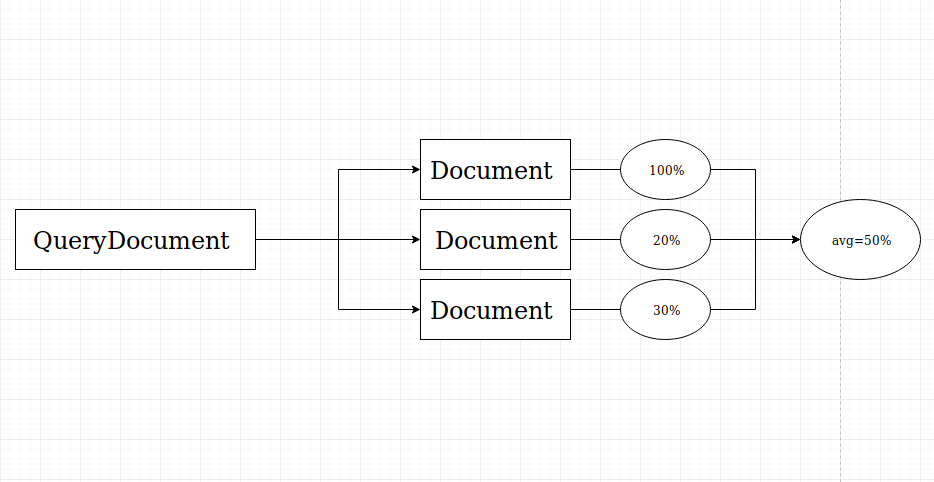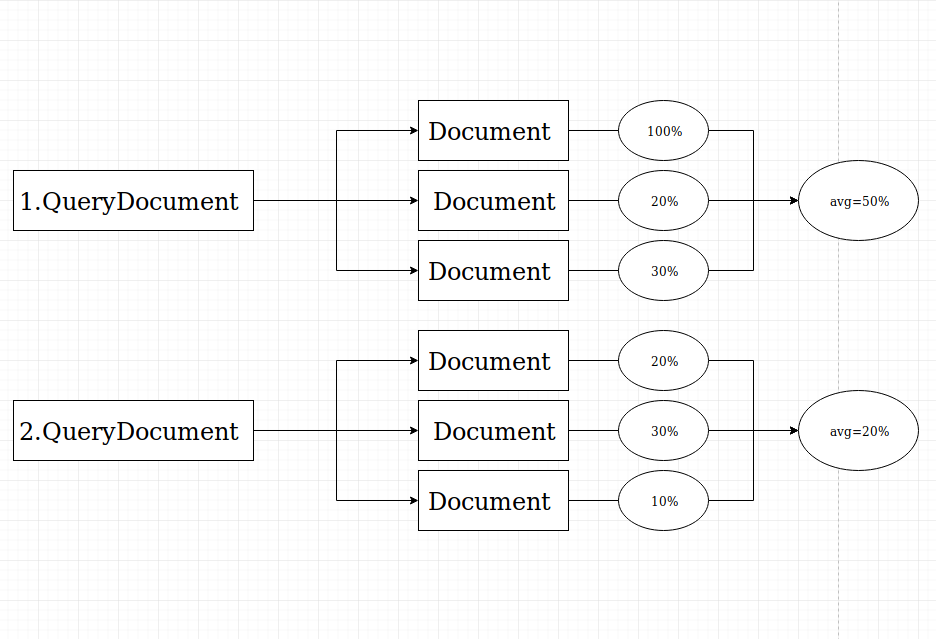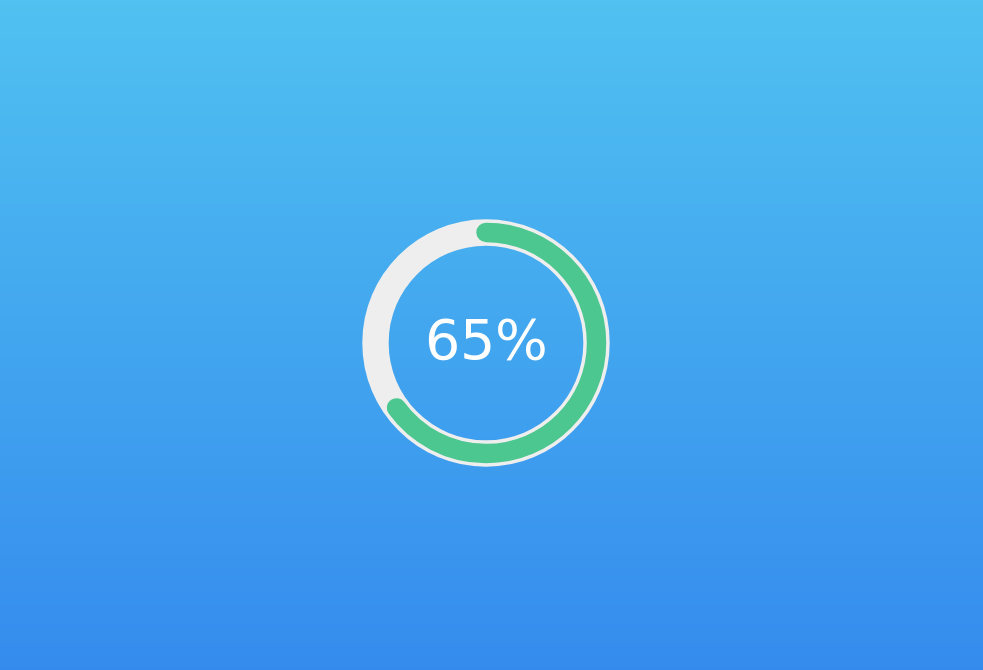## Compare documents similarity using Python | NLP

Hey Reverse PY Network! In this post we are going to build a web application which will compare the similarity between two documents. We will learn the very basics of natural language processing (NLP) which is a branch of artificial intelligence that deals with the interaction between computers and humans using the natural language.

Let's start with the base structure of program but then we will add graphical interface to making the program much easier to use. Feel free to contribute this project in my GitHub.

#### NLTK and Gensim

Natural language toolkit (NLTK) is the most popular library for natural language processing (NLP) which was written in Python and has a big community behind it. NLTK also is very easy to learn, actually, it’ s the easiest natural language processing (NLP) library that we are going to use. It contains text processing libraries for tokenization, parsing, classification, stemming, tagging and semantic reasoning.

Gensim is billed as a Natural Language Processing package that does ‘Topic Modeling for Humans’. But it is practically much more than that. It is a leading and a state-of-the-art package for processing texts, working with word vector models (such as Word2Vec, FastText etc)

Topic models and word embedding are available in other packages like scikit, R etc. But the width and scope of facilities to build and evaluate topic models are unparalleled in gensim, plus many more convenient facilities for text processing. Another important benefit with gensim is that it allows you to manage big text files without loading the whole file into memory.

First, let's install nltk and gensim by following commands:

```pip install nltk
pip install gensim
```

#### Tokenization of words (NLTK)

We use the method word_tokenize() to split a sentence into words. Take a look example below

```from nltk.tokenize import word_tokenize

data = "Mars is approximately half the diameter of Earth."
print(word_tokenize(data))
```

Output:

```['Mars', 'is', 'approximately', 'half', 'the', 'diameter', 'of', 'Earth']
```

#### Tokenization of sentences (NLTK)

An obvious question in your mind would be why sentence tokenization is needed when we have the option of word tokenization. We need to count average words per sentence, so for accomplishing such a task, we use sentence tokenization as well as words to calculate the ratio.

```from nltk.tokenize import sent_tokenize

data = "Mars is a cold desert world. It is half the size of Earth. "
print(sent_tokenize(data))
```

Output:

```['Mars is a cold desert world', 'It is half the size of Earth ']
```

Now, you know how these methods is useful when handling text classification. Let's implement it in our similarity algorithm.

#### Open file and tokenize sentences

Create a .txt file and write 4-5 sentences in it. Include the file with the same directory of your Python program. Now, we are going to open this file with Python and split sentences.

```import nltk
from nltk.tokenize import word_tokenize, sent_tokenize

file_docs = []

with open ('demofile.txt') as f:
for line in tokens:
file_docs.append(line)

print("Number of documents:",len(file_docs))
```

Program will open file and read it's content. Then it will add tokenized sentences into the array for word tokenization.

#### Tokenize words and create dictionary

Once we added tokenized sentences in array, it is time to tokenize words for each sentence.

```gen_docs = [[w.lower() for w in word_tokenize(text)]
for text in file_docs]
```

Output:

```[['mars', 'is', 'a', 'cold', 'desert', 'world', '.'], ['it', 'is', 'half', 'the', 'size', 'of', 'earth', '.']]
```

In order to work on text documents, Gensim requires the words (aka tokens) be converted to unique ids. So, Gensim lets you create a Dictionary object that maps each word to a unique id. Let's convert our sentences to a [list of words] and pass it to the corpora.Dictionary() object.

```dictionary = gensim.corpora.Dictionary(gen_docs)
print(dictionary.token2id)
```

Output:

```{'.': 0, 'a': 1, 'cold': 2, 'desert': 3, 'is': 4, 'mars': 5, 'world': 6, 'earth': 7, 'half': 8, 'it': 9, 'of': 10, 'size': 11, 'the': 12}
```

A dictionary maps every word to a number. Gensim lets you read the text and update the dictionary, one line at a time, without loading the entire text file into system memory.

#### Create a bag of words

The next important object you need to familiarize with in order to work in gensim is the Corpus (a Bag of Words). It is a basically object that contains the word id and its frequency in each document (just lists the number of times each word occurs in the sentence).

Note that, a ‘token’ typically means a ‘word’. A ‘document’ can typically refer to a ‘sentence’ or ‘paragraph’ and a ‘corpus’ is typically a ‘collection of documents as a bag of words’.

Now, create a bag of words corpus and pass the tokenized list of words to the Dictionary.doc2bow()

Let's assume that our documents are:

```Mars is a cold desert world. It is half the size of the Earth.
```

```corpus = [dictionary.doc2bow(gen_doc) for gen_doc in gen_docs]
```

Output:

```{'.': 0, 'a': 1, 'cold': 2, 'desert': 3, 'is': 4,
'mars': 5, 'world': 6, 'earth': 7, 'half': 8, 'it': 9,
'of': 10, 'size': 11,'the': 12}
[[(0, 1), (1, 1), (2, 1), (3, 1), (4, 1), (5, 1), (6, 1)],
[(0, 1), (4, 1), (7, 1), (8, 1), (9, 1), (10, 1), (11, 1), (12, 2)]]
```

As you see we used "the" two times in second sentence and if you look word with id=12 (the) you will see that its frequency is 2 (appears 2 times in sentence)

#### TFIDF

Term Frequency – Inverse Document Frequency(TF-IDF) is also a bag-of-words model but unlike the regular corpus, TFIDF down weights tokens (words) that appears frequently across documents.

Tf-Idf is calculated by multiplying a local component (TF) with a global component (IDF) and optionally normalizing the result to unit length. Term frequency is how often the word shows up in the document and inverse document frequency scales the value by how rare the word is in the corpus. In simple terms, words that occur more frequently across the documents get smaller weights.

```This is the space. This is our planet. This is the Mars.
```

```tf_idf = gensim.models.TfidfModel(corpus)
for doc in tf_idf[corpus]:
print([[dictionary[id], np.around(freq, decimals=2)] for id, freq in doc])
```

Output:

```[['space', 0.94], ['the', 0.35]]
[['our', 0.71], ['planet', 0.71]]
[['the', 0.35], ['mars', 0.94]]
```

The word ‘the’ occurs in two documents so it weighted down. The word ‘this’ and 'is' appearing in all three documents so removed altogether.

#### Creating similarity measure object

Now, we are going to create similarity object. The main class is Similarity, which builds an index for a given set of documents.The Similarity class splits the index into several smaller sub-indexes, which are disk-based. Let's just create similarity object then you will understand how we can use it for comparing.

``` # building the index
sims = gensim.similarities.Similarity('workdir/',tf_idf[corpus],
num_features=len(dictionary))
```

We are storing index matrix in 'workdir' directory but you can name it whatever you want and of course you have to create it with same directory of your program.

#### Create Query Document

Once the index is built, we are going to calculate how similar is this query document to each document in the index. So, create second .txt file which will include query documents or sentences and tokenize them as we did before.

```file2_docs = []

with open ('demofile2.txt') as f:
for line in tokens:
file2_docs.append(line)

print("Number of documents:",len(file2_docs))
for line in file2_docs:
query_doc = [w.lower() for w in word_tokenize(line)]
query_doc_bow = dictionary.doc2bow(query_doc) #update an existing dictionary and
create bag of words
```

We get new documents (query documents or sentences) so it is possible to update an existing dictionary to include the new words.

#### Document similarities to query

At this stage, you will see similarities between the query and all index documents. To obtain similarities of our query document against the indexed documents:

```# perform a similarity query against the corpus
query_doc_tf_idf = tf_idf[query_doc_bow]
# print(document_number, document_similarity)
print('Comparing Result:', sims[query_doc_tf_idf])
```

Cosine measure returns similarities in the range <-1, 1> (the greater, the more similar).

Assume that our documents are:

```Mars is the fourth planet in our solar system.
It is second-smallest planet in the Solar System after Mercury.
Saturn is yellow planet.
```

and query document is:

```Saturn is the sixth planet from the Sun.
```

Output:

```[0.11641413 0.10281226 0.56890744]
```

As a result, we can see that third document is most similar

#### Average Similarity

What's next? I think it is better to calculate average similarity of query document. At this time, we are going to import numpy to calculate sum of these similarity outputs.```import numpy as np

sum_of_sims =(np.sum(sims[query_doc_tf_idf], dtype=np.float32))
print(sum_of_sims)
```

Numpy will help us to calculate sum of these floats and output is:

```# [0.11641413 0.10281226 0.56890744]
0.78813386
```

To calculate average similarity we have to divide this value with count of documents

```percentage_of_similarity = round(float((sum_of_sims / len(file_docs)) * 100))
print(f'Average similarity float: {float(sum_of_sims / len(file_docs))}')
print(f'Average similarity percentage: {float(sum_of_sims / len(file_docs)) * 100}')
print(f'Average similarity rounded percentage: {percentage_of_similarity}')
```

Output:

```Average similarity float: 0.2627112865447998
Average similarity percentage: 26.27112865447998
Average similarity rounded percentage: 26
```

Now, we can say that query document (demofile2.txt) is 26% similar to main documents (demofile.txt)

What if we have more than one query documents?

As a solution, we can calculate sum of averages for each query document and it will give us overall similarity percentage.Assume that our main document are:

```Malls are great places to shop, I can find everything I need under one roof.
I love eating toasted cheese and tuna sandwiches.
Should we start class now, or should we wait for everyone to get here?
```

By the way I am using random word generator tools to create these documents. Anyway, our query documents are:

```Malls are goog for shopping. What kind of bread is used for sandwiches? Do we have to start class now, or should we wait for
everyone to come here?
```

Let's see the code:

````python
avg_sims = [] # array of averages

# for line in query documents
for line in file2_docs:
# tokenize words
query_doc = [w.lower() for w in word_tokenize(line)]
# create bag of words
query_doc_bow = dictionary.doc2bow(query_doc)
# find similarity for each document
query_doc_tf_idf = tf_idf[query_doc_bow]
# print (document_number, document_similarity)
print('Comparing Result:', sims[query_doc_tf_idf])
# calculate sum of similarities for each query doc
sum_of_sims =(np.sum(sims[query_doc_tf_idf], dtype=np.float32))
# calculate average of similarity for each query doc
avg = sum_of_sims / len(file_docs)
# print average of similarity for each query doc
print(f'avg: {sum_of_sims / len(file_docs)}')
# add average values into array
avg_sims.append(avg)
# calculate total average
total_avg = np.sum(avg_sims, dtype=np.float)
# round the value and multiply by 100 to format it as percentage
percentage_of_similarity = round(float(total_avg) * 100)
# if percentage is greater than 100
# that means documents are almost same
if percentage_of_similarity >= 100:
percentage_of_similarity = 100
```

Output:

```Comparing Result: [0.33515707 0.02852172 0.13209888]
avg: 0.16525922218958536
Comparing Result: [0.         0.21409164 0.27012902]
avg: 0.16140689452489218
Comparing Result: [0.02963242 0.         0.9407785 ]
avg: 0.3234703143437703
```

We had 3 query documents and program computed average similarity for each of them. If we calculate these values result will:

```0.6501364310582478
```

We are formatting the value as percentage by multiplying it with 100 and rounding it to make a value simpler. The final result with Django:#### Mission Accomplished!

Great! I hope you learned some basics of NLP from this project. In addition, I implemented this algorithm in Django for create graphical interface. Feel free to contribute project in my GitHub.

I hope you learned something from this lab 😃 and if you found it useful, please share it and join me on social media! As always Stay Connected!🚀

Instagram